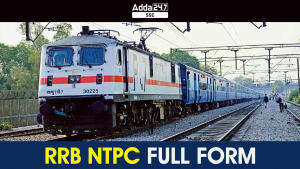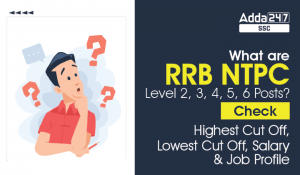Latest SSC jobs   »   RRB NTPC Mathematics Quiz : 13th...

# RRB NTPC Mathematics Quiz : 13th November

Q1. If a BSES electricity bill is paid from Paytm before due date, one gets a reduction of 4% on the amount of the bill, by paying a bill before due date a person got a reduction of Rs 13. The amount of his electricity bill was:
(a) 125
(b) 225
(c) 325
(d) 425

Q2. Vinugopal decided to donate 5% of his July’s salary on the day of donation he changed his mind and donated 1687.50, which was 75% of what he had decided earlier. How much is Vinugopal’s salary?
(a) 45000
(b) 50000
(c) 44000
(d) 40000

Q3. A Raymond’s Trader marked the selling price of an article at 10% above the cost price. At the same time of selling he allowed certain discount and suffers a loss of 1%. He allowed a discount of:
(a) 11%
(b) 10%
(c) 9%
(d) 10.5%

Q4. Six-elevenths of a number is equal to 22% of second number. Second number is equal to the one-fourth of third number. The value of the third number is 2400. What is the 45% of the first number?
(a) 108.9
(b) 111.7
(c) 117.6
(d) None of these

Q5. In an entrance examination Rockie scored 56% marks, perry scored 92% marks and Srifi scored 634 marks. The maximum marks of the examination are 875, what are the average mark scored by all the three girls together:
(a) 929
(b) 815
(c) 690
(d) 643

Q6. A diligent man was engaged on a job for 40 days on the condition that he will get a wage of Rs. 180 for the day he works, but he will have to pay a fine of Rs. 20 for each day of his absence. If he gets Rs. 5200 at the end of the 40 days, then, he was absent for how many days?
(a) 12 days
(b) 10 days
(c) 6 days
(d) 8 days

Q7. How many kg of pure salt must be added to 40 kg of 4% solution of salt and water to increase it to 12% solution of mixture ?
(a) 2.04 kg
(b) 2.64 kg
(c) 3.14 kg
(d) 3.64 kg

Q8. The average of the essay-I test scores of a class of ‘x’ students is 80 and that of ‘y’ student is 94. When the scores of both the classes are combined, the average becomes 86. What is the ratio of x to y?
(a) 6 : 5
(b) 5 : 4
(c) 4 : 3
(d) 7 : 6

Q9. The ages of Angaad, Bankie and Cherry together are 57 years. Bankie is thrice as old as Angaad and Cherry is 12 years older than Angaad. Then, the respective age of Angaad, Bankie and Cherry is:
(a) 8, 26, 23 (years)
(b) 9, 27, 21 (years)
(c) 8, 25, 24 (years)
(d) 9, 26, 22 (years)

Q10. If 35% of a number is subtracted from the second number the second number reduces to its four–fifths. What is the ratio the first number to the second number?
(a) 4 : 7
(b) 4 : 9
(c) 5 : 9
(d) 5 : 7

solutions:-S6.Ans(b)
Sol.
Let the number of days he was absent be x days.
180 (40 – x) – 20 x = 5200
7200 – 180x – 20x = 5200
7200 – 200x = 5200
x = 2000/200 = 10 days

S7.Ans(d)
Sol.
Amount of salt in 40 kg of solution = 4/100 * 40 = 1.6 kg
Let x kg of pure salt be added.
Then, (1.6 + x)/ (40 + x) = 12/100
160 + 100x = 480 + 12x
88x = 320
x = 3.64 kg

S8.Ans(c)
Sol.
(80x + 94y)/(x + y) = 86
80x + 94y = 86x + 86y
8y = 6x
x/y = 8/6 = 4/3 = 4 : 3

S9.Ans(b)
Sol.
Let the age of Angad be x years
Bankie’s age = 3x
Cherry’s age = x + 12
x + 3x + x + 12 = 57
or, 5x + 12 = 57
x = 9
Angad, Bankie and Cherry’s age are 9, 27, 21 years

S10.Ans(a)
Sol.
N2 – 35% of N1 = 4/5 N2
N2 – 4/5N2 = 35/100N1
N2/5 = 35/100N1
N1/N2 = 1/5 * 100/35 = 4 : 7

Important Links for RRB NTPC Recruitment 2019

#### Congratulations!General Awareness & Science Capsule PDFIncorrect details? Fill the form again here

General Awareness & Science Capsule PDF

Thank You, Your details have been submitted we will get back to you.

### TOPICS:

•RRB NTPC Exam Pattern 2023, Check Comple...
•RRB NTPC Selection Process 2023 CBT 1, C...
•RRB NTPC Full Form: All You Need To Know
•Railways Senior Commercial cum Ticket Cl...
•RRB NTPC CBT 2 Syllabus 2023, Topics, Su...
•What are RRB NTPC Level 2, 3, 4, 5, 6 Po...